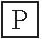# User Forum

Subject :IMO    Class : Class 7

If '+' stands for '× ', '-' stands for '÷', '× ' stands for '-', ÷ stands for '+', then value of P is .6 - 2 + 8 × 12 ÷ 3 >A15
B18
C21
D14

why is this not 15 ?

Class : Class 7

## Ans 2:

Class : Class 7
15 is right but the question says what number is less then 15 , so it is 14. Hence the correct answer is D.14

## Ans 3:

Class : Class 8
Answer is 15 because 6÷2×8-1+3 =3×8 =24-12=12 12+3=15

Class : Class 7